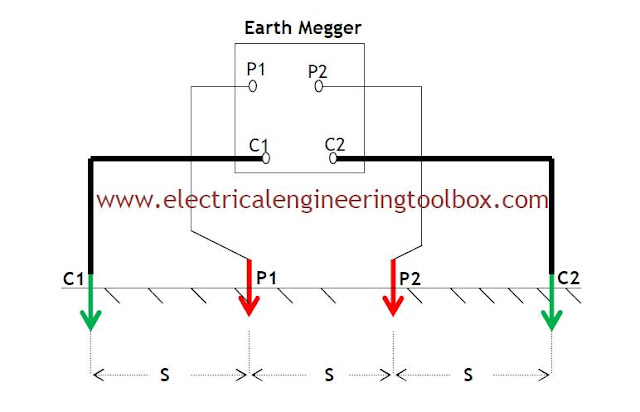### How to Measure Soil Resistivity

Custom Search
Wenner’s four-electrode method is popularly used to measure soil resistivity. In this method Four electrodes are driven into the earth along a straight line at equal intervals, S. The depth of the electrodes in the ground is always of the order of 10 to 15 cm. The earth megger is placed on a steady and approximately level base. The four electrodes are connected to the earth megger terminal as shown below:A current, I , is passed through the two outer electrodes C1 & C2 and earth. A voltage difference V, is observed between the two inner electrodes PI & P2. The current I flowing into the earth produces an electric field proportional to its density and to the resistivity of the soil. The voltage V measured between the inner electrodes is, therefore, proportion to the field. Consequently, the resistivity will be proportional to the ratio of the voltage to the current, i.e., R.
The soil resistivity is then calculated as:

Where:
ρ =  Soil Resistivity of soil in Ohms-meter
S = distance between two successive electrodes in    meters
R = ratio of voltage to current or electrode resistance in Ohms
e = depth of burial of electrode in the ground
If the separation distance of the electrodes , S, is much greater than the depth of burial, e, of electrodes, then equation 1 above reduces to :
Modern equipment used for testing for soil resistivity use the Wenner four electrode system and the mathematical calculation above, is done automatically by these new set of equipment such that once setup properly, at the press of a button, the value of the soil resistivity is determined quickly.